# How to create a computable drop-down list

Excel
Dynamic, dependable, conditional, or calculated drop-down list in Excel implies that the contents of the drop-down list for the current cell should depend on values in the other cell and change dynamically when the value of the controlling cell changes.

For example, you need to select gifts for the employee's children from the lists for different ages and have a spreadsheet with:

• Name and birth date of the child
• Age of the child calculated the following formula (see How to calculate age in Excel) for the 1st of August as the current date:

= DATEDIF (<start date>, TODAY (), "y")

where TODAY () returns the current date, "y" tells DATEDIF to count full years between <start date> and TODAY ():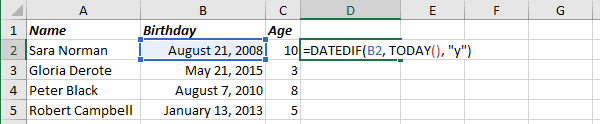• The next column should contain drop-down lists that show different gift options depending on the calculated age, for example: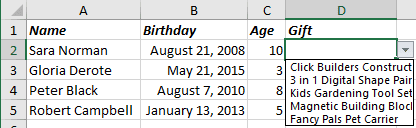To create the dynamic or computable drop-down list, do the following:

1.   For every set of options for the dependent drop-down list, create a named range (see Creating and using named ranges), for example:

• First – for children from 1 to 5 years
• Second – for children from 5 to 9 years
• Third – for children under 9 years: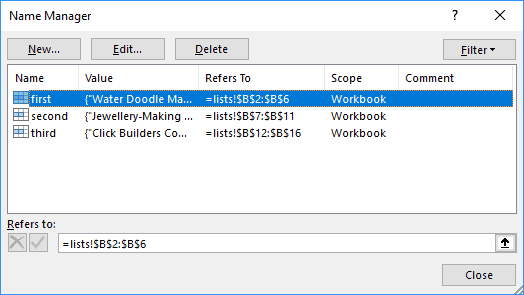2.   Add a drop-down list (see Creating a drop-down list in a cell):

2.1.   On the Data tab, in the Data Tools group, click Data Validation:2.2.   In the Data Validation dialog box, on the Settings tab: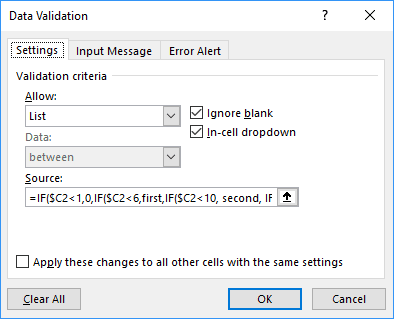• In the Allow drop-down list, select List.
• In the Source box, specify the range, using the nested IF formula:

= IF (\$C2<1, 0, IF (\$C2<6, first, IF (\$C2<10, second, IF (\$C2<17, third,))))

or the shorter formula using IFS added in Excel 2016:

= IFS (\$C2<1, 0, \$C2<6, first, \$C2<10, \$C2<17, third).

• Make sure to check the In-Cell Dropdown option.

Now, Excel will show different lists for different ages: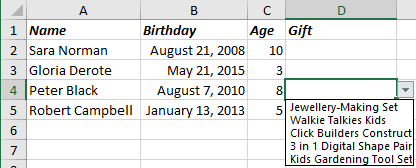Note: If you need an automatically extended drop-down list in Excel that automatically adds new items to the list of choices, check the tip How to create an automatically extended or dynamic drop-down list.

See also this tip in French: Comment créer une liste déroulante calculable.